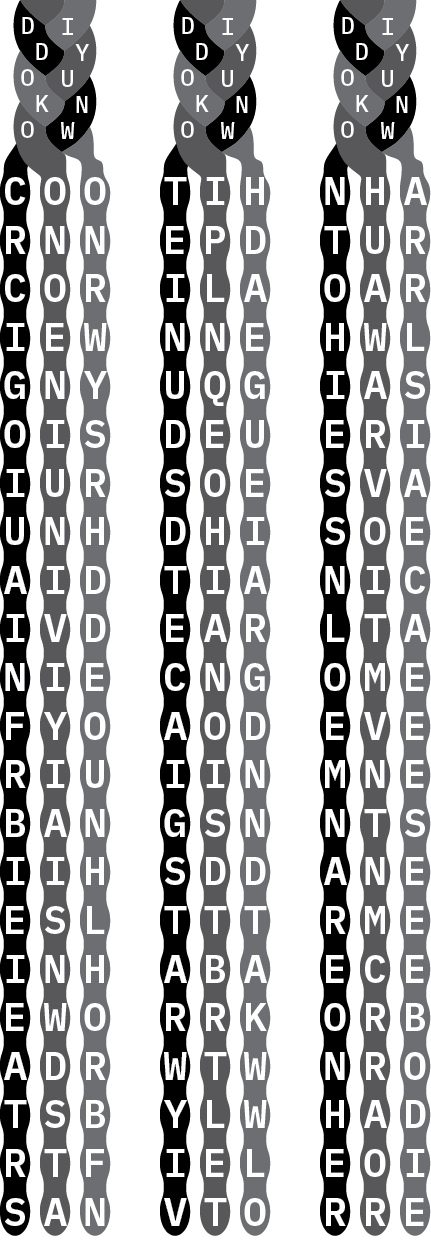National Museum of African American History and Culture

Errata - Jan 2 1:45 PM EST: In a previous version, the image was inconsistent with the copy-able text. The image has been fixed.

The social meaning encoded into braids’ multiple layers brings with it centuries of accumulated information, beliefs, and practices. Braiding operates as a bridge spanning the distance between the past, present, and future. It creates a tangible, material thread connecting people often separated by thousands of miles and hundreds of years.Copy-able Text

 d i d i d i d y d y d y o u o u o u k n k n k n o w o w o w
 c o o t i h n h a r n n e p d t u r c o r i l a o a r i e w n n e h w l g n y u q g i a s o i s d e u e r i i u r s o e s v a u n h d h i s o e a i d t i a n i c i v d e a r l t a n i e c n g o m e f y o a o d e v e r i u i i n m n e b a n g s n n t s i i h s d d a n e e s l t t t r m e i n h a b a e c e e w o r r k o r b a d r w t w n r o t s b y l w h a d r t f i e l e o i s a n v t o r r e